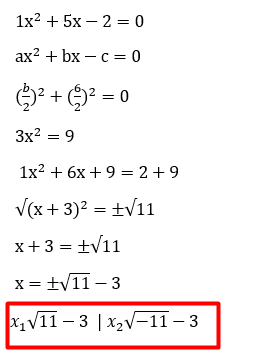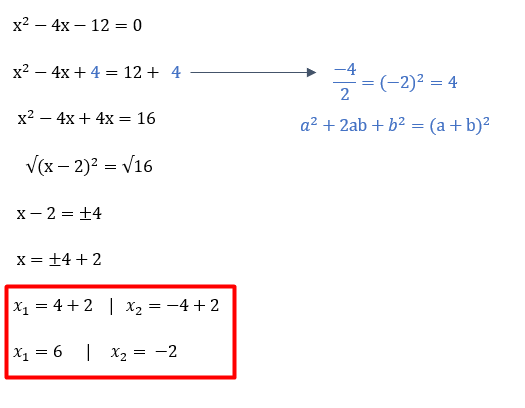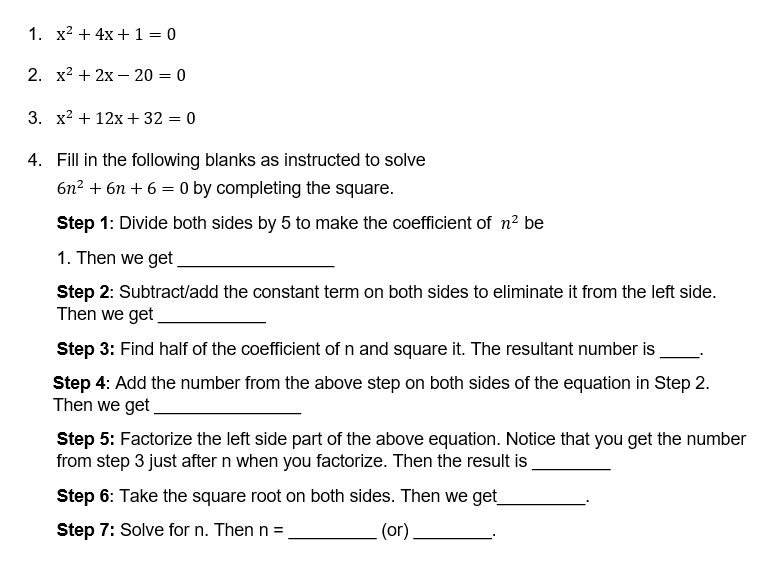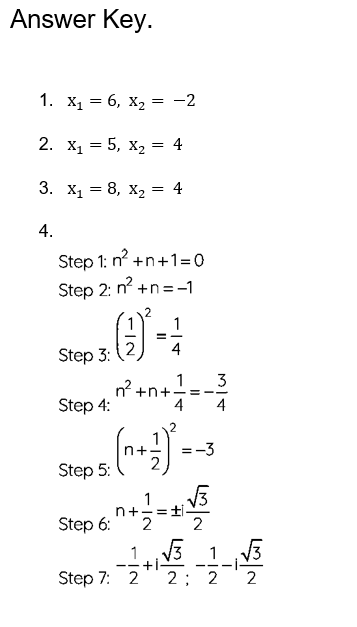# Solving Quadratic Equations by Completing the Square Worksheet Algebra 1 (with answer key)

Students can better understand algebra by solving quadratic equations by filling in the square worksheets. These simple math problems are designed to help students develop their understanding of quadratic expressions. The student gains proficiency with the more basic ideas, the teacher introduces more challenging questions based on finding roots, solving quadratic equations, and others.

What is the “Solving Quadratic Equations by Completing the Square Worksheet Algebra 1”?

This worksheet will explore some of the methods for solving quadratic equations by completing the square.

## What is Completing Square?

One method for locating the roots of a quadratic equation is to use the square method. We must solve the given equation as a perfect square using this method. Using the quadratic formula, we can also assess the quadratic equation’s roots.

This worksheet offers visual simulations that aid in understanding the subject at hand. They are flexible, simple to use, and completely free to download.

Instructions on how to use the “Solving Quadratic Equations by Completing the Square Worksheet Algebra 1.”

Study the concept and examples given and try to solve the given exercises below.

## Conclusion

Students can develop their ability to quickly calculate by working through the problems in worksheets on quadratic equations. Additionally, they gain an understanding of a number of related ideas that employ quadratic equations. Additionally, a student will be certain to receive high marks by practicing a variety of problems at various levels of difficulty.

If you have any inquiries or feedback, please let us know.

## Solving Quadratic Equations by Completing the Square Worksheet Algebra 1 (with answer key)

Completing the square

Manipulate your equation so you end up with one squared part that equals a number.

Example A:Example B:## Solve.